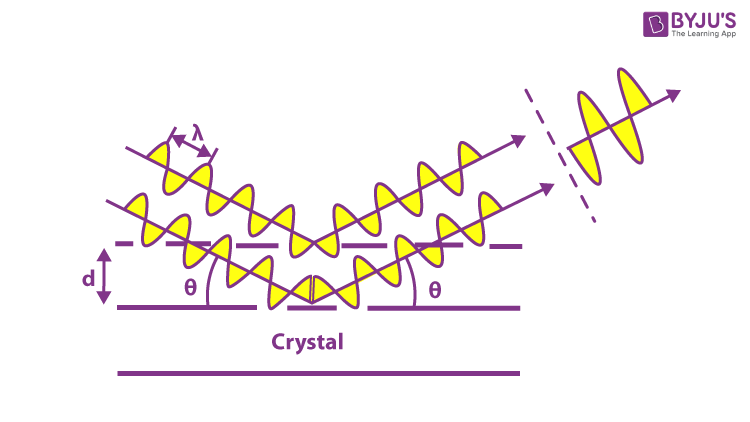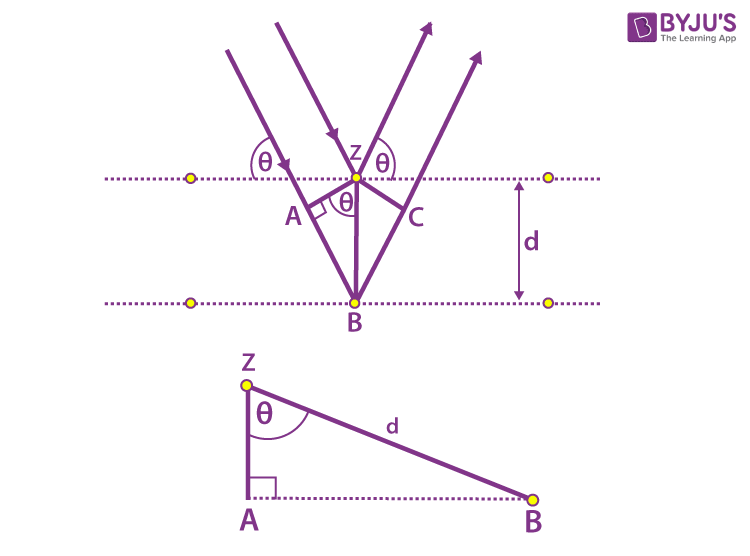Checkout JEE MAINS 2022 Question Paper Analysis : Checkout JEE MAINS 2022 Question Paper Analysis :

# Bragg's Law

We have studied electromagnetic waves and the properties of x-rays in our previous sessions. Let us know what happens when the X-ray is incident onto a crystal surface by learning Bragg’s Law. This law helps understand coherent and incoherent scattering from a crystal lattice. Let us know about Bragg’s law, Bragg equation, Bragg’s derivation and its applications.

## What is Bragg’s Law?

Bragg’s law is a special case of Laue diffraction, which determines the angles of coherent and incoherent scattering from a crystal lattice. When X-rays are incident on a particular atom, they make an electronic cloud move like an electromagnetic wave. The movement of these charges radiates waves again with similar frequency, slightly blurred due to different effects, and this phenomenon is known as Rayleigh scattering. Basically, this law explains the relationship between an x-ray light shooting and its reflection from a crystal surface.

Brag’s Law states the following:

When the X-ray is incident onto a crystal surface, its angle of incidence, θ, will reflect with the same angle of scattering, θ. And, when the path difference, d is equal to a whole number, n, of wavelength, λ, constructive interference will occur.

The exact process takes place upon scattering neutron waves via nuclei or a coherent spin interaction with an isolated electron. These wavefields that are re-emitted interfere with each other destructively or constructively, creating a diffraction pattern on a film or detector. The diffraction analysis is the resulting wave interference, and this analysis is known as Bragg diffraction.

### Bragg Equation

According to Bragg Equation:

nλ = 2d sinΘ

Therefore, according to the equation of Bragg’s Law:

• The equation explains why the faces of crystals reflect X-ray beams at particular angles of incidence (Θ, λ).
• The variable d indicates the distance between the atomic layers, and the variable The variable d indicates the distance between the atomic layers, and the variable λ specifies the wavelength of the incident X-ray beam and n as an integer.This observation illustrates the X-ray wave interface, called X-ray diffraction (XRD) and proof of the atomic structure of crystals.

Bragg was also awarded the Nobel Prize in Physics for identifying crystal structures starting with NaCl, ZnS, and diamond. In addition, to understand the structure of every state of matter by any beam, e.g., ions, protons, electrons, neutrons, with a wavelength similar to the length between the molecular structures, diffraction was developed.

### Derivation of Bragg’s Law

Consider the following figure of beams in which the phases of the beams coincide when the incident angle is equal to the reflecting angle. The incident beams are parallel to each other until they reach point z. When they are at point z, they strike the surface and travel upwards. At point B, the second beam scatters. AB + BC is the distance travelled by the second beam. The extra distance is known as the integral multiple of the wavelength.nλ = AB + BC

We also know that AB = BC

nλ = 2AB (equation 1)

d is the hypotenuse of the right triangle Abz. Ab is the opposite of the angle θ.

AB = d sinθ (equation 2)

Substituting equation 2 in equation 1

nλ = 2d sinθ

The above equation is Bragg’s law expression.

 Related Topics:

## Applications of Bragg’s Law

There are numerous applications of Bragg’s law in the field of science. Some common applications are given in the points below.

• In the case of XRF (X-ray fluorescence spectroscopy) or WDS (Wavelength Dispersive Spectrometry), crystals of known d-spacings are used for analyzing crystals in the spectrometer.
• In XRD (X-ray diffraction) the inter-planar spacing or d-spacing of a crystal is used for characterization and identification purposes.

### Bragg’s Diffraction

Bragg’s diffraction was first proposed by William Henry Bragg and William Lawrence Bragg, in 1913. Bragg’s diffraction occurs when a subatomic particle or electromagnetic radiation waves have wavelengths that are comparable to atomic spacing in a crystal lattice.

### Solved Examples

Example 1:

The wavelength of the X-rays is 0.071 nm which is diffracted by a plane of salt with 0.28 nm as the lattice constant. Determine the glancing angle for the second-order diffraction. Assume the value of the salt plane to be 110, and the given salt is rock salt.

Solution:

Given:

Wavelength of the X-rays = 0.071 nm

Lattice constant = 0.28 nm

Plane = 110

Order of diffraction = 2

Glancing angle =?

Using Bragg’s law:

2d sin Ө = nλ

Rock salt has FCC, therefore,

$$\begin{array}{l}d=\frac{a}{\sqrt{h^{2}+k^{2}+l^{2}}}\end{array}$$

Substituting the values,

$$\begin{array}{l}d=\frac{0.28\times 10^{-9}}{\sqrt{1^{2}+1^{2}+0^{2}}}=\frac{0.28\times 10^{-9}}{\sqrt{2}}m\end{array}$$

Substituting in the Bragg’s equation,

Ө = 21°

Example 2:

The wavelengths of first-order X-rays are 2.20 Å at 27°8’. Find the distance between the adjacent Miller planes.

Solution:

Using Bragg’s law,

2d sin Ө = nλ

Where,

n = 1

λ = 2.20 Å

Ө = 27°8’

Substituting the values, we get

d = 2.20 Å

## Bragg’s law Conclusion

The concluding ideas from Bragg’s law are:

• The diffraction has three parameters i.e, the wavelength of X rays,λ
• The crystal orientation defined by the angle θ
• The spacing of the crystal planes, d.

The diffraction can be considered to occur for a given wavelength and set of planes. For instance, changing the orientation continuously, i.e., changing θ until Bragg’s Law is satisfied.## Frequently Asked Questions – FAQs

### What is Bragg’s Law?

When the X-ray is incident onto a crystal surface, its angle of incidence, θ, will reflect with the same angle of scattering, θ. And, when the path difference, d is equal to a whole number, n, of wavelength λ, constructive interference will occur.

### What does Bragg’s Law tell us?

Bragg’s law explains the relationship between an x-ray light shooting and its reflection from a crystal surface.

### Why is Bragg’s Law important?

Bragg law is useful for measuring wavelengths and for determining the lattice spacings of crystals.

### What are the applications of Bragg’s Law?

In the case of XRF (X-ray fluorescence spectroscopy) or WDS (Wavelength Dispersive Spectrometry), crystals of known d-spacings are used for analyzing crystals in the spectrometer.

### Bragg’s law experiment is based on which scattering of waves?

Bragg’s law experiment is based on the Rayleigh scattering in which the charges are scattered without a change in their wavelength.

### What is an X-ray?

An X-ray is a penetrating energetic electromagnetic radiation which has a wavelength between 10 nanometers and 10 picometers.

### Who developed Bragg’s law?

Lawrence Bragg developed Bragg’s law.

### What is meant by the scattering of light?

Scattering of light occurs when light rays are scattered by particles in the medium.

### What is Bragg’s equation?

Bragg’s equation is nλ = 2d sinΘ.

### Who proposed Bragg’s diffraction?

Lawrence Bragg and William Henry Bragg proposed Bragg’s diffraction.

Stay tuned to BYJU’S and Fall in Love with Learning!

Test your knowledge on Braggs Law

1. S Deepika

Nice